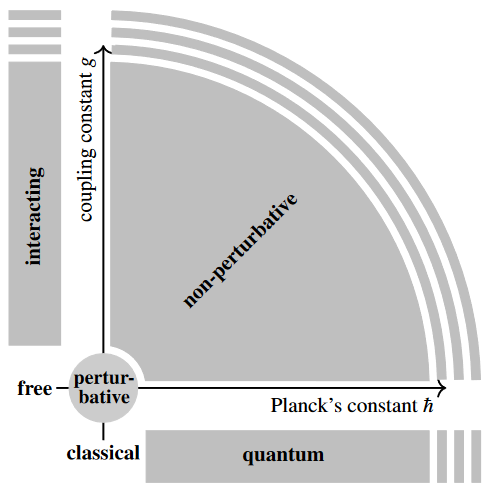# nLab non-perturbative effect

Contents

### Context

#### Quantum field theory

functorial quantum field theory

# Contents

## Idea

An effect in non-perturbative quantum field theory that cannot be seen in perturbative quantum field theory is called a non-perturbative effect.

More in detail, theories with instanton field configurations (such as in Yang-Mills theory, hence in QCD and QED) or branes (such as in string theory), etc., are expected to have observables which as functions of the coupling constant $g$ are transseries of the form

(1)$Z(g) = \sum_n a_n g^n + e^{-A/g} \sum_n a_n^{(1)} g^n + e^{-B/g^2} \sum_n b_n^{(1)} g^n \,,$

where the first sum is the Feynman perturbation series itself and where the terms with a non-analytic dependence of the form $\exp(-A/g)$ or $\exp(-A/g^2)$ are the contributions of the instantons. Since all the derivatives of the functions $g \mapsto e^{-1/g}$ or $g \mapsto e^{-1/g^2}$ vanish at coupling constant $g = 0$, the Taylor series of this part of the observable does not appear in perturbative QFT, even though it is present. Therefore this is called a non-perturbative effect.

Related is resurgence theory. See also at perturbation theory – Divergence/convergence for more.

Notice that, while non-perturbative effects are generally the least understood, they are not exotic but ubiquitous: Essentially the entire parameter space of a generic “physical theory” is non-perturbative. In fact, the non-perturbative region of parameter space is the complement of an infinitesimally thickened point (labeled “perturbative” in the following graphics):## Examples

### Confinement and the mass gap

A central example of a non-perturbative effect is confinement (hence the “mass gap problem”) in Yang-Mills theory at low temperature. Perturbation theory is not suited to explain this (e.g Espiru 94, section 7).

### Quark-gluon plasma

At the other extreme of high temperature QCD, also the quark-gluon plasma, while now deconfined is thought to be strongly coupled.

### Hadronic physics in flavour and $g-2$ anomalies

Non-perturbative effects in hadron-physics affects the discussion of possible beyond-standard model physics as seen in

### Worldsheet and brane instantons in string/M-theory

Consider the M-theory scales

• $\ell_P$, the Planck length in 11-dimensions;

• $R_{10}$ the length (circumference) of the $S^1_{10}$ circle fiber for KK-compactification to 10 dimensions

and the string theory scales

Then under the duality between M-theory and type IIA string theory these scales are related as follows:

$\ell_P \;=\; g_{st}^{1/3} \ell_s \,, \phantom{AAA} R_{10} \;=\; g_{st} \ell_s$

equivalently

$\ell_s \;=\; R_{10}/ g_{st} \,, \phantom{AAA} \ell_P \;=\; g_{st}^{-2/3} R_{10}$

equivalently

$g_{st} \;=\; (R_{10}/\ell_P)^{3/2} \,, \phantom{AAA} \ell_s \;=\; \ell_P (R_{10}/\ell_P)^{-1/2} \,.$

Hence a membrane instanton, which on a 3-cycle $C_3$ gives a contribution

$\exp\left( - \frac{ vol(C_3) }{ \ell^3_P } \right)$

becomes

1. if the cycle wraps, $C_3 = C_2 \cup S^1_{10}$, a worldsheet instanton

$\exp\left( - \frac{ vol(C_3) }{ \ell_P^3 } \right) \;=\; \exp\left( - \frac{ R_{10} vol(C_2) }{ g_{st} \ell_s^3 } \right) \;=\; \exp\left( - \frac{ vol(C_2) }{ \ell_s^2 } \right)$
2. the cycle does not wrap, a spacetime instanton contribution, specifically a D2-brane instanton?

$\exp\left( - \frac{ vol(C_3) }{ \ell_P^3 } \right) \;=\; \exp\left( - \frac{ vol(C_3)/\ell_s^3 }{ g_{st} } \right)$

(This unification of the two different non-perturbative effects in perturbative string theory (worldsheet instantons and spacetime instantons), to a single type of effect (membrane instanton) in M-theory was maybe first made explicit in Becker-Becker-Strominger 95. Brief review includes Marino 15, sections 1.2 and 1.3).

### In field theory

Discussion of traditional algebraic quantum field theory as being about non-perturbative effects (and therefore lacking any interesting models in 3+1 dimensions, so far…):

Genral introduction and toy examples (e.g. phi^4-theory or anharmonic oscillator):

Discussion for phi^4 theory is in

• Marco Serone, from 2:46 on in A look at $\phi^4_2$ using perturbation theory, January 2018 (recording)

Discussion for QCD includes

• Marcos Mariño, Instantons and Large $N$ – An introduction to non-perturbative methods in QFT (pdf)

and in (super-)Yang-Mills theory and string theory is in

### In phenomenology

• Ulrich Nierste, Flavour Anomalies: Phenomenology and BSM Interpretations, 2018 (pdf)

• Fred Jegerlehner, The Role of Mesons in Muon $g-2$ (arXiv:1809.07413)

In cosmology:

### In string theory

The form of the contribution of non-perturbative effects in string theory was originally observed in

• Stephen Shenker, The Strength of nonperturbative effects in string theory, presented at the Cargese Workshop on Random Surfaces, Quantum Gravity and Strings, Cargese, France, May 1990 (spire)

The interpretation via D-branes of non-perturbative effects in the string coupling constant is due to

The identification of non-perturbative contributions in string theory with brane contributions is due to

Review includes

Reviews specifically in type II string theory include

• Hugo Looyestijn, Non-perturbative effects in type IIA string theory, Master Thesis 2006 (pdf)

• Angel Uranga, Non-perturbative effects and D-brane instanton resummation in string theory (pdf, pdf)

### In M-Theory

The relation of non-perturbative effects in string theory to M-theory goes back to

In Becker-Becker-Strominger 95 it was realized that the worldsheet instantons and D-brane instantons of string theory unify to membrane instantons (see Marino 15, section 1.3)